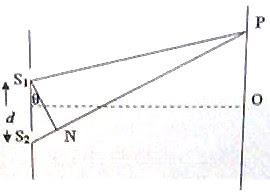## Sunday, September 28, 2008

### IIT-JEE 2008 Question (MCQ) on Young’s Double Slit

Today we will discuss two questions on Young’s double slit. The following multiple correct answers type question appeared in IIT-JEE 2008 question paper:

In a Young’s double slit experiment, the separation between the two slits is d and the wave length of the light is λ. The intensity of light falling on slit 1 is four times the intensity of light falling on slit 2. Choose the correct choice(s).

(A) If d = λ, the screen will contain only one maximum.

(B) If λ < d < 2λ, at least one more maximum (besides the central maximum) will be observed on the screen.

(C) If the intensity of light falling on slit 1 is reduced so that it becomes equal to that of slit 2, the intensities of the observed dark and bright fringes will increase.

(D) If the intensity of light falling on slit 2 is reduced so that it becomes equal to that of slit 1, the intensities of the observed dark and bright fringes will increaseThe path difference [S2N = (S2P S1P) in fig.] between the interfering beams is d sinθ. Therefore for obtaining a maximum at the point P on the screen the condition to be satisfied is

dsinθ =

When d = λ, sinθ = n

Since sinθ ≤ 1, n ≤ 1

The possible values of n are 0 and 1. But the maximum corresponding to n = 1 cannot be observed since θ cannot be 90º. Therefore, the central maximum of order zero corresponding to zero path difference (n = 0) alone can be observed. Option A is therefore correct

If λ < d < 2λ, the limiting values of d are λ and 2λ. In addition to the usual central maximum, fringes of order up to n satisfying the equation, dsinθ = will be obtained, where d < 2λ.

If d = 2λ, we have 2λ = nλ/sinθ so that n = 2 sinθ.

Since sinθ should be less than 1 for observing the fringe, the maximum value of n must be 1. Therefore, the central maximum and the maximum of order n = 1 will be observed. Option B too is therefore correct

Options C and D are obviously incorrect since the intensity of the dark fringes will become zero when the light beams passing through the slits are of equal intensity.

Now consider another question on Young’s double slit:

Suppose the wave length λ and the double slit separation d in a Young’s double slit experiment are such that the 6th dark fringe is obtained at point P shown in the above figure. The path difference (S2P – S1P) will be

(a) 5 λ

(b) 5 λ/2

(c) 6 λ

(d) 3 λ

(e) 11 λ/2

The dark fringe of order 1 (1st dark fringe) is formed when the path difference is λ/2. The dark fringe of order 2 is formed when the path difference is 3λ/2 (and not 2λ/2) and the dark fringe of order 3 is formed when the path difference is 5λ/2 (and not 3λ/2). Generally, the dark fringe of order n is formed when the path difference is (2n 1)λ/2. The 6th dark fringe is therefore formed when the path difference is 11λ/2.

By clicking on the label ‘wave optics’ below this post, you can access all related posts on this site.

You will find useful posts on wave optics here at apphysicsresources.